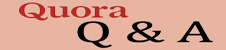## MECHANISM OF GRAVITYGravity was found by Sir Issac Newton by 17th century but 'Mechanism behind Gravity' remained unexplained so far. The technical paper explains the mechanism of gravity by introduction of a novel concept that 'Gravity is born due to a FM wave through the space medium'.

The author has proved the wave basis causation of Gravity by a mathematical derivation of Newton's equation of Gravity.